## Practical Multi-Touch DisplayAs one of the first graphing calculator, the HP Prime offers a 3.5-inch, multi-touch-enabled color screen that reacts to touch with your finger, which means all features of the HP Prime can be activated by briefly tapping the screen. HP presents a calculator with a convenient operating system, many people are already familiar with smartphones or tablets. The benefits of multi-touch technology can be seen in many ways:

• Graphs or charts can be moved for further analysis by a finger gesture. This eliminates the tedious navigating through control keys.
• By touching the screen geometric constructions can be produced as on a drawing sheet. The tensile mode can be executed directly with a finger movement in all directions.
• The keys of the calculator are complemented by appropriate menu fields at the bottom of the display appear depending on the context. By touching a displayed menu field, the corresponding function is executed.

On the HP Prime it is possible to add text files (in an HP format). A search is not possible, so students will have to scroll through the entire document. Erasing the memory, however, removes these documents so that they no longer be used during exams and tests. This applies to all user-added content .

## Clear operating conceptThe HP Prime is equipped with a clear operating concept. This consists of three basic components, that can be opened with the keys Apps, Home and CAS.

In the Apps environment, a variety of applications are available that can make tasks visualized and solved from a variety of mathematical and scientific disciplines.
All apps are equally controlled by the Symb, Plot and Num control keys.

• Apps-Environment
• Home-Environment
• In the home environment, the HP Prime behaves like an ordinary calculator, which means the results are calculated numerical
• CAS-Environment
• In the CAS environment, the Computer Algebra System is active, which means all calculations will be processed symbolic.

## Numeric Home EnvironmentIn the home environment, all numbers are numerically interpret and all calculations executed numerically. For the calculations both the "text book" input mode and the HP-typical "RPN" input mode are available.

Over one hundred mathematical functions are already available directly from the keyboard.
Many other math functions are in the Math Menu, that has been divided in several sections:

• Numbers:
Rounding numbers.
• Arithmetic:
Calculate maximum, minimum, dividing radicals and percentages.
• Complex numbers:
Calculating real part, imaginary, conjugated, arguments and unit vectors.
• Trigonometric Functions:
CSC, ACSC, SEC, ASEC, COT, ACOT.
• Hyperbolic Functions:
SINH, ASINH, COSH, ACOSH, TANH, ATANH.
• Probability:
calculating faculties, combinations, permutations, random numbers and of probabilities for normal, binomial or Poisson distributions.
• Lists and matrices:
Creating lists (or matrices) and execute functions on these lists (or matrices).

## Symbolic CAS EnvironmentIn the CAS environment, all figures and all arithmetic operations are interpreted symbolically .

Some of the over one hundred CAS functions are already available directly from the keyboard. Many other features are found in CAS menu , which is divided into different sections:

• Algebra:
Simplify, summarize, multiplying, factoring, dismantling of arithmetic expressions.
• Analysis:
Form of derivatives, integrals, differential operators, limits, sums, Laplace and Fourier transforms.
• Solve:
Solving equations and differential equations.
• Converting:
Apply power and trigonometric principles to calculate expressions.
• Number theory:
Divider, gcd, lcm, prime factorization, prime number, Euclidean algorithm modulo calculation.
• Polynomials:
Zeros, dividers, factorization, gcd, lcm, Euclidean algorithm, Groebner basis, hermite, Lagrange, Laguerre, Legendre, Chebyshev polynomials.
• Graphing:
Functions in one and two variables, implicit functions, direction fields, isolines.

## DATALOGGING ON HP PRIME: HP STREAMSMART 410

The HP StreamSmart 410 helps math and science students visualize experiment results by streaming data in real time. Make experiments come alive with the HP StreamSmart 410.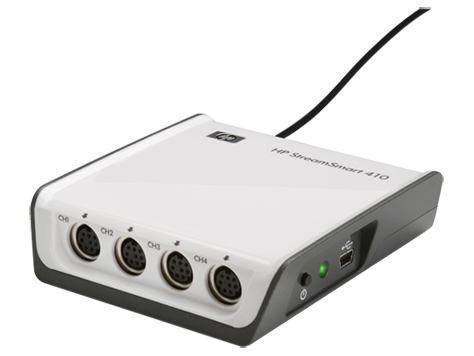Designed for use in math and science classes, the StreamSmart 410 connects real-world data to a student’s graphing calculator, with accurate and immediate real-time streaming. Suited for use in a wide range of subjects, including: math, science, pre-algebra, algebra, trigonometry/pre-calculus, calculus, statistics, earth science, environmental science, physical science, physics, biology, and chemistry.

No waiting around to see results
• Watch and learn with real-time data collection as it occurs. Learning becomes exciting when you can capture up to four streams of data (motion, sound, temperature, light, etc.) and simultaneously see the results in real time – without any delays!
• Easily collect data points at rates of 5,000 or more samples per second1
• Identifies sensor type automatically and selects unit of measurement and optimal scaling
• Zoom in or out on the data streams in real time
• Supports data streaming, data logging, selection of events, and events with entry

Use with HP Prime Graphing Calculators (G8X92AA) and Fourier data sensors
• Designed for use with the HP Prime Graphing Calculator (G8X92AA) and Fourier measurement sensors
• Export selected data to the HP Prime Graphing Calculator (G8X92AA) for further analysis

Set up experiments quickly and easily
• Solution is small and ultra-portable
• Automatically sends the collected data to the built-in analysis tool. No need to learn a new set.

## Powerful Plotting

To show the capacity of the plotting power on HP Prime, you can access a plot-gallery with several large functions. Here some of the examples: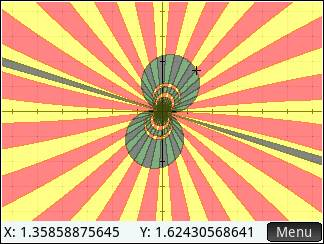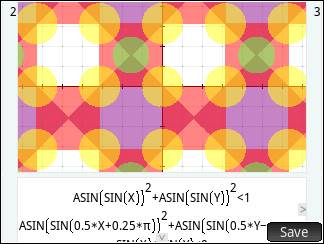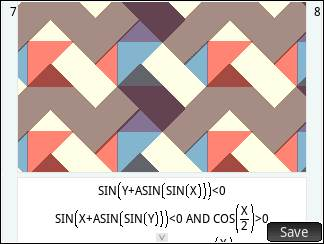1.       Open the advanced Graphing App

2.       Enter X=Y

3.       Press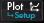4.       Press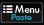5.       Choose option 5: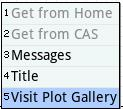## Wireless communication

The wireless kit** enables communication between teacher / examiner and student . It works similarly to a wireless mouse : the student chooses the network of his choice and then connects automatically . So, when several teacher-kits are available, this means that the correct network has to be chosen for a new teacher once. If they have the same teacher every week , they just have to do this once, a next time/lesson, the Prime will automatically connect .  The choice of networks is very simple , by pressing an icon in the corner of the screen and choose the right network.Getting your HP Prime Wireless Kit set up for classroom use is quick and easy. Please follow these steps to get started.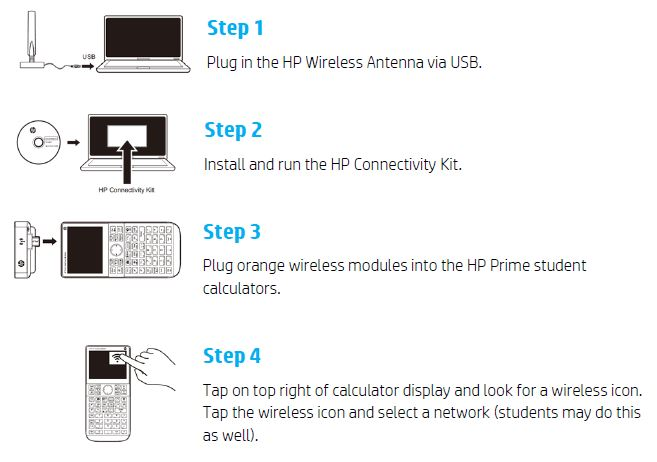**Wireless Kit needs to be purchased separately